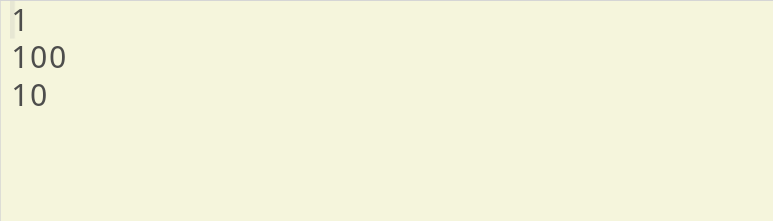# Scope of Variables in C

By now we've known how to declare a variable, but what comes along with it is the scope of a variable. In this post, we'll find out about Scope of Variables in C.Scope of Variables in C

## What is Scope of a Variable?

Scope here refers to a particular portion of an entire program or a part of program. Someone we even define scope as a region in any program. But when it is said together with variables; like variable scope or scope of variables then it refers to area of that program where the variables can be used after its declaration.

Clearly inferring that a scope of a variable is that region within which a variable can be accessed but out of that region, the variable can't be used anymore. Previously when we discussed about functions, you might have noticed that any variable declared inside a function can't be called outside of it. Hence, we can draw a conclusion that there exists different types of scope of variables. Let's check them one by one.

## Types of Scopes of Variables

Based on position of any variable we can classify their scope in following manner:

PositionType
Inside a function or a block.Local variables
Out of all functions.Global variables
In the function parameters.Formal parameters

### Local Variables

When a variable is declared within a function block like below:

``````#include <stdio.h>
void test()
{
/*local variable of function test*/
int x = 10;
printf("%d\n",x);
}
int main()
{
/*local variable of function main*/
int x = 100;
{
/*local variable of this block*/
int x = 1;
printf("%d\n",x);
}
printf("%d\n",x);
test();
}``````

#### Output:Local Variable Scope in C

Then the variable can be accessed only within the function block, in that case the variable is a local variable and such variables have a local scope.

Likewise in the above sample code, the value of the variable ‘x’ is 1 in the block of code ({ }) defined inside the ‘main’ function and the value of this variable ‘x’ in the ‘main’ function outside this block of code ({ }) is 100. The value of this variable ‘x’ is 10 in the function ‘test’.

### Global Variables

Those variables which are defined outside of all functions are referred to as global variable. Once declared, these variables can be accessed by any function in that program. Thus, such variables are said to have a global scope. If we see the code below:

``````#include <stdio.h>
/*Global variable*/
int x = 1000;
void test2()
{
/*local variable of same name*/
int x = 100;
printf("%d\n",x);
}

int main()
{
printf("%d\n",x);
test2();
}``````

#### Output:Global Variable Scope in C

Here, you can see that the value of the local variable ‘x’ was given priority inside the function ‘test2’ over the global variable have the same name ‘x’.

The following table gives you details about how global variables are defined based on data-type.

datatypeInitial Default Value
int0
char'\0'
float0
double0
pointerNULL

### Formal Parameters

The parameters which are used inside the body of a function are referred to as formal parameters. Formal parameters are treated as local variables in that function and are prioritised over the global variables.

``````#include <stdio.h>
/*Global variable*/
int x = 100;
void test3(int x)
{
/*x is a formal parameter*/
printf("%d\n",x);
}

int main()
{
test3(10);
}``````

#### Output:Formal Variable Scope in C

All programs written in this post are compiled online.

### Books I Prefer:I hope this article was helpful to understand different variable scopes in C! Comment below, if you've got any question. Head back soon for another interesting article on C Programming.